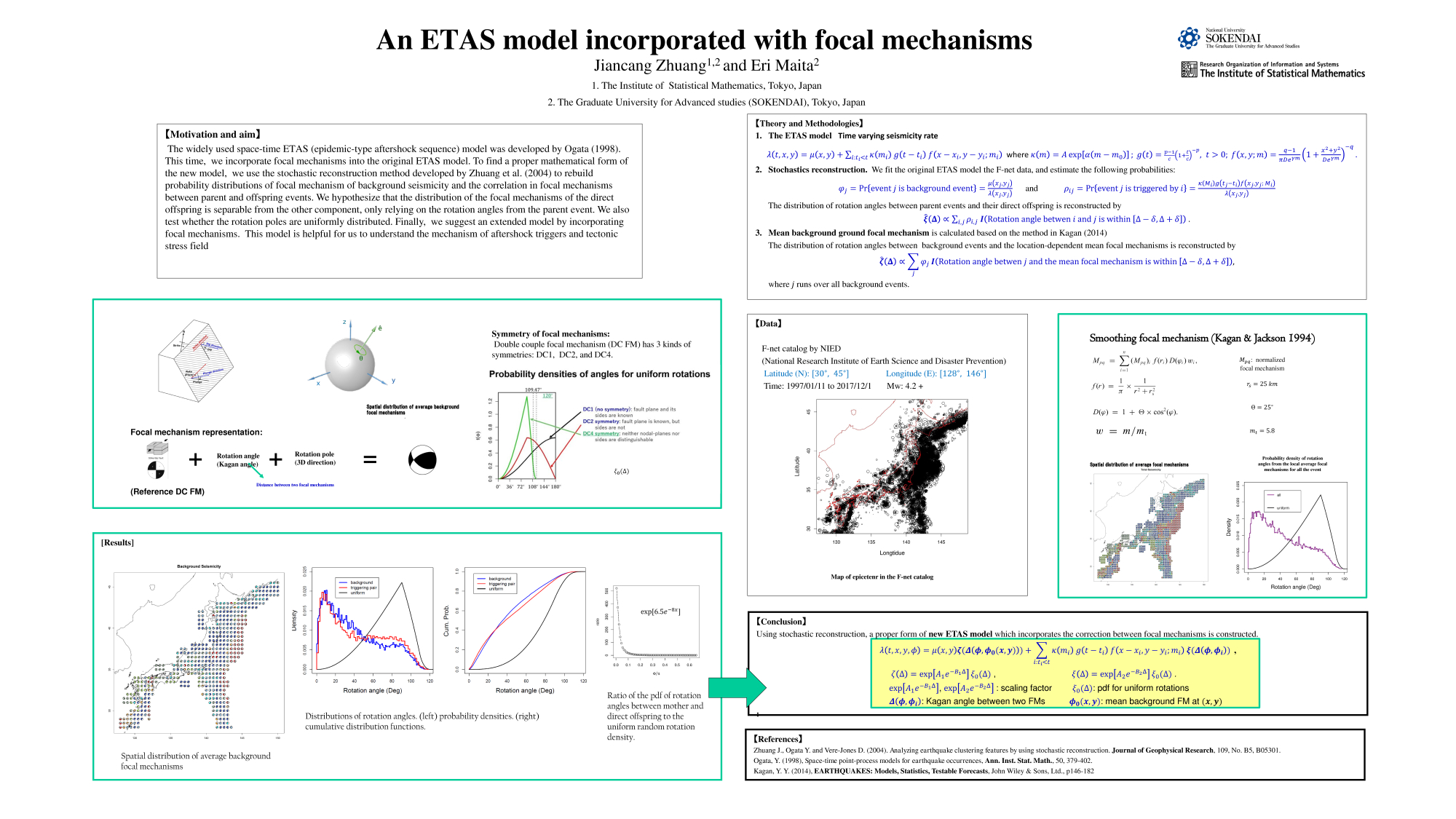## Poster #101, Earthquake Forecasting and Predictability (EFP)

### An ETAS model incorporated with focal mechanisms

Poster Image:#### Poster Presentation

2020 SCEC Annual Meeting, Poster #101, SCEC Contribution #10446
This study tries to find the probability distributions of focal mechanisms in background seismicity and earthquake clusters in Japan by analyzing F-net data. To describe the probability distribution, each focal mechanism is discomposed as the result of a rotation about a particular pole starting from a reference focal mechanism. In this study, DC4 symmetry is considered, i.e., either nodal-plane can be the fault plane and the two sides of the fault plane are distinguishable. To separate the background and clustering seismicity, we fit the space-time ETAS model (e.g., Zhuang, 2011, EPS) to the earthquakes with magnitude 4.2+ from 1997 to 2017 in the F-net catalog, and use the stochastic declu...stering method to estimate the background probabilities that each event is a background event and the triggered probabilities that each event is triggered by other events.

For the background events, the reference focal mechanism is taken as the average focal mechanism in each area, calculated by the method in Kagan and Jackson (2014, GJI). The ration angles between a background event and the mean focal mechanisms at (x, y) is reconstructed by histogram of rotation angles between the each earthquake, weighted by its background probability estimated from the ETAS model, and local mean focal mechanism. For the triggered events, the reference focal mechanism is naturally taken the focal mechanism of the event which triggers it. The rotation angles between the parent event and its direct offspring are also reconstructed by histogram of rotation angles between each triggering pair, weighted by the probability that the 2nd event is triggered by the first event in the pair. We also find that the rotation poles are almost uniformly distributed.

We then plug the reconstructed focal mechanisms distribution into the space-time ETAS model and apply it to the F-net data. The results show that focal mechanism plays an important role in earthquake triggering and incorporation with focal mechanism greatly improves model fitting.